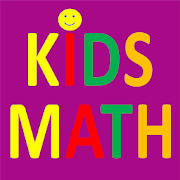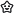# Kids Math: Multiply, Divide, Add, Subtract fun wayEveryoneAges 6-12
291Kids Math, provides free and fun filled Cool Mental Math games for Elementary kids for practicing Addition, Subtraction, Multiplication, and Division. It helps you to increase your Math calculation speed and accuracy. This game is most suitable for kids from age 7 to 12 years. Also, adults who love math will enjoy this game. It may be challenging for grown ups too.

There are total 20 free educational games for kids to play and practice addition, subtraction, multiplication, and division ( +,-,/,*). There are total 12 levels for each game. Each level has 10 questions. First four levels are untimed. You are required to do all 10 questions correctly to move to the next level. Levels from 5 to 12 are timed levels. You are required to answer all questions correctly within the specified time. As the level progresses the time to achieve the level goes down.

Addition games include addition questions for the numbers from 0 to 999. Below are the details of the addition math games-
- Single Digit Addition: It includes the sum questions for the numbers from 0 to 9.
- Two-digit Addition without Regrouping: It includes the sum questions involving the numbers from 0 to 99. All addition questions in this game are without regrouping. They don't require carry over.
- Three digit Addition without Regrouping: It includes the sum questions involving the numbers from 0 to 999. All addition questions in this game are without regrouping. They don't require carry over.
-Two-digit Additional with Regrouping: It includes the sum questions for numbers from 0 to 99. Addition questions in this addition game include regrouping.

Subtraction games include subtraction questions for the numbers from 0 to 99. Below are details of the subtraction math games-
- Single digit Subtraction: it includes the subtraction question for the numbers from 0 to 9
- Subtraction up to 99: It includes the subtraction questions for the numbers from 0 to 99. This game does not include any regrouping requiring carryover.
- Subtraction up to 999: It includes the subtraction questions for the numbers from 0 to 999. This subtraction game does not include any regrouping requiring carry over
- Subtraction up to 99: With Regrouping - it includes the subtraction questions for the numbers from 0 to 99. This subtraction game includes the subtraction questions needing regrouping.

Multiplication Games include total 8 multiplication games. Below are details of Multiplication math games-
- Multiplication - up to 5x10: It includes the multiplication questions up from the multiplication tables up to 5 x 10.
- Multiplication - up to 10x10: It includes the multiplication questions up from the multiplication tables up to 10 x 10.
- Multiplication - up to 15x10: It includes the multiplication questions up from the multiplication tables up to 15 x 10.
- Multiplication - up to 20x10: It includes the multiplication questions up from the multiplication tables up to 20 x 10.
- Multiplication - up to 5x20: It includes the multiplication questions up from the multiplication tables up to 5 x 20.
- Multiplication - up to 10x20: It includes the multiplication questions up from the multiplication tables up to 10 x 20.
- Multiplication - up to 15x20: It includes the multiplication questions up from the multiplication tables up to 15 x 20.
- Multiplication - up to 20x20: It includes the multiplication questions up from the multiplication tables up to 20 x 20.

Division games include 4 division games. Below are the details of division math games-
- Division - up to 20/10: It includes the division questions with numerator up to 20 and denominator up to 10
- Division - up to 50/10: It includes the division questions with numerator up to 50 and denominator up to 10
- Division - up to 100/10: It includes the division questions with numerator up to 100 and denominator up to 10
- Division - up to 400/20: It includes the division questions with numerator up to 400 and denominator up to 20
Collapse

Review Policy
4.0
291 total
5
4
3
2
1

Collapse

Updated
October 25, 2019
Size
7.6M
Installs
50,000+
Current Version
3.0
Requires Android
5.0 and up
Content Rating
Everyone
Permissions
Offered By
AAlearning